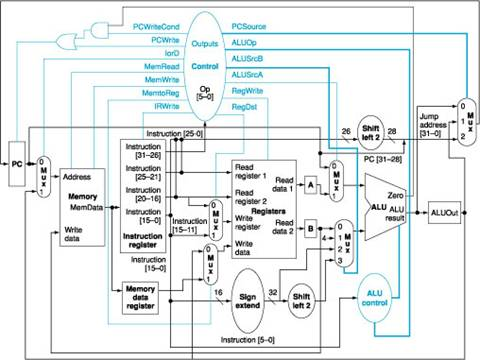Computer Organization and Structure

Homework #4

Due: 2006/12/26

1.      Describe the effect that a single stuck-at-n fault (i.e., regardless of what it should be, the signal is always n, where n = 0 or 1) would have for the signals in the following sub-questions:

a.         For single stuck-at-0 fault, which instructions, if any, will not work correctly in the single-cycle datapath as shown in Figure 1? Explain why. Consider each of the following faults separately:

a)      RegWrite = 0

b)      ALUop0 = 0

c)      ALUop1 = 0

d)     Branch = 0

f)       MemWrite = 0Figure 1: The simple datapath with the control unit.

b.        For single stuck-at-1 fault, which instructions, if any, will not work correctly in the single-cycle datapath? Explain why.

c.         For single stuck-at-0 fault, which instructions, if any, will not work correctly in the multiple-cycle datapath as shown in Figure 2? Explain why. Consider each of the following faults separately:

a)      RegWrite = 0

c)      MemWrite = 0

d)     IRWrite = 0

e)      PCWrite = 0

f)       PCWriteCond = 0Figure 2: The multicycle datapath with the control lines.

d.        For single stuck-at-1 fault, which instructions, if any, will not work correctly in the multiple-cycle datapath? Explain why.

2.      Two important parameters control the performance of a processor: cycle time and cycles per instruction. There is an enduring trade-off between these two parameters in the design process of microprocessors. While some designers prefer to increase the processor frequency at the expense of large CPI, other designers follow a different school of thought in which reducing the CPI comes at the expense of lower processor frequency. Consider the following machines, and compare their performance using the following instruction mix: 25% loads, 13% stores, 47% ALU instructions, and 15% branches/jumps.

M1: The multicycle datapath is designed as shown in Figure 3 with a 1 GHz clock.

M2: A machine like M1 except that register updates are done in the same clock cycle as a memory read of ALU operation. Thus in Figure 4, states 6 and 7 and states 3 and 4 are combined. This machine has an 3.2 GHz clock, since the register update increases the length of the critical path.

M3: A machine like M2 except that effective address calculations are done in the same clock cycle as a memory access. Thus states 2, 3, and 4 can be combined, as can 2 and 5, as well as 6 and 7. This machine has a 2.8 GHz clock because of the long cycle created by combining address calculation and memory access.

Find out which of the machines is fastest. Are there instruction mixes that would make another machine faster, and if so, what are they?Figure 3: The complete datapath for the multicycle implementation together with the necessary control lines.Figure 4: The complete finite state machine control for the datapath shown in Figure 2.